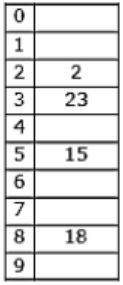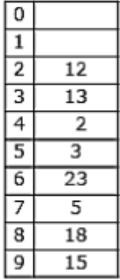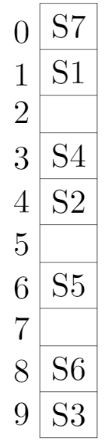## Hashing

 Question 1

Which one of the following hash functions on integers will distribute keys most uniformly over 10 buckets numbered 0 to 9 for i ranging from 0 to 2020?

 A h(i) = i2 mod 10 B h(i) = i3 mod 10 C h(i) = (11 *i2) mod 10 D h(i) = (12 * i) mod 10
Data-Structures       Hashing       GATE 2015 [Set-2]
Question 1 Explanation:
If we take first 10 elements, number of collisions taken by the hash function given by option (B) is less when compared to others.
 Question 2

Given a hash table T with 25 slots that stores 2000 elements, the load factor α for T is ___________.

 A 80 B 70 C 60 D 50
Data-Structures       Hashing       GATE 2015 [Set-3]
Question 2 Explanation:
Load factor(α) = no. of elements/no. of slots = 2000/25 = 80
 Question 3

Consider a hash table with 9 slots. The hash function is h(k) = k mod 9. The collisions are resolved by chaining. The following 9 keys are inserted in the order: 5, 28, 19, 15, 20, 33, 12, 17, 10. The maximum, minimum, and average chain lengths in the hash table, respectively, are

 A 3, 0, and 1 B 3, 3, and 3 C 4, 0, and 1 D 3, 0, and 2
Data-Structures       Hashing       GATE 2014 [Set-1]
Question 3 Explanation:
Hash table has 9 slots.
h(k) = k mod 9
Collisions are resolved using chaining.
Keys: 5, 28, 19, 15, 20, 33, 12, 17, 10.5%9 – 5
28%9 – 1
19%9 – 1
15%9 – 6
20%9 – 2
33%9 – 6
12%9 – 3
17%9 – 8
10%9 – 1
Maximum chain length is 3
Minimum chain length is 0
Average chain length = 0+3+1+1+0+1+2+0+1/ 9 = 1
 Question 4

Consider a hash table with 100 slots. Collisions are resolved using chaining. Assuming simple uniform hashing, what is the probability that the first 3 slots are unfilled after the first 3 insertions?

 A (97×97×97)/1003 B (99×98×97)/1003 C (97×96×95)/1003 D (97×96×95)/(3!×1003)
Algorithms       Hashing       GATE 2014 [Set-3]
Question 4 Explanation:
Given Hash table consists of 100 slots.
They are picked with equal probability of Hash function since it is uniform hashing.
So to avoid the first 3 slots to be unfilled
= 97/100*97/100*97/100 =((97*97*97))⁄1003
 Question 5

A hash table of length 10 uses open addressing with hash function h(k)=k mod 10, and linear probing. After inserting 6 values into an empty hash table, the table is as shown below.How many different insertion sequences of the key values using the same hash function and linear probing will result in the hash table shown above?

 A 46, 42, 34, 52, 23, 33 B 34, 42, 23, 52, 33, 46 C 46, 34, 42, 23, 52, 33 D 42, 46, 33, 23, 34, 52
Data-Structures       Hashing       GATE 2010
Question 5 Explanation:
Hash Table consists of 10 slots, uses Open Addressing with hash function and linear probing.
After inserting 6 values, the table looks likeThe possible order in which the keys are inserted are:
34, 42, 23, 46 are at their respective slots 4, 2, 3, 6.
52, 33 are at different positions.
― 52 has come after 42, 23, 34 because, it has the collision with 42, because of linear probing, it should have occupy the next empty slot. So 52 is after 42, 23, 34.
― 33 is after 46, because it has the clash with 23. So it got placed in next empty slot 7, which means 2, 3, 4, 5, 6 are filled.
42, 23, 34 may occur in any order but before 52 & 46 may come anywhere but before 33.So option (C)Question 6

A hash table of length 10 uses open addressing with hash function h(k)=k mod 10, and linear probing. After inserting 6 values into an empty hash table, the table is as shown below.How many different insertion sequences of the key values using the same hash function and linear probing will result in the hash table shown above?

 A 10 B 20 C 30 D 40
Data-Structures       Hashing       GATE 2010
Question 6 Explanation:
Total 6 insertions
― 33 must be inserted at last (only one possibility)
― 46 can be inserted in any of the 5 places remaining. So 5 ways.
― 52 must be inserted only after inserting 42, 23, 34. So only one choice for 52.
〈42,23,34〉 can be sequenced in 3! ways.
Hence, 5×3! = 30 ways
 Question 7

The keys 12, 18, 13, 2, 3, 23, 5 and 15 are inserted into an initially empty hash table of length 10 using open addressing with hash function h(k) = k mod 10 and linear probing. What is the resultant hash table?

 ABCDData-Structures       Hashing       GATE 2009
Question 7 Explanation:
Given keys: 12, 18, 13, 2, 3, 23, 5 & 15
They are inserted into an initially empty hash table of length 10 using open addressing with hash function h(k)=k mod 10 & linear probing is used.12 % 10 = 2
18 % 10 = 8
13 % 10 = 3
2 % 10 = 2 (Index 4 is empty)
3 % 10 = 3 (Index 5 is empty)
23 % 10 = 3 (Index 6 is empty)
5 % 10 = 5 (Index 7 is empty)
15 % 10 = 5 (Index 9 is empty)
Hence Option C is correct.
A & B doesn’t include all the keys & option D is similar to chaining. So, will go with C.
 Question 8

Consider a hash table of size seven, with starting index zero, and a hash function (3x + 4) mod 7. Assuming the hash table is initially empty, which of the following is the contents of the table when the sequence 1, 3, 8, 10 is inserted into the table using closed hashing? Note that ‘_’ denotes an empty location in the table.

 A 8, _, _, _, _, _, 10 B 1, 8, 10, _, _, _, 3 C 1, _, _, _, _, _,3 D 1, 10, 8, _, _, _, 3
Data-Structures       Hashing       GATE 2007
Question 8 Explanation:
Consider a hash table of size 7
Starting index is zero i.e.,⇾ Given hash function is = (3x+4) mod 3
⇾ Given sequence is = 1, 3, 8, 10
where x = 1 ⟹ (3(1)+4)mod 3 = 0
1 will occupy index 0.
where x = 3 ⟹ (3(3)+4) mod 7 = 6
3 will occupy index 6.
where x = 8 ⟹ (3(8)+4) mod 7 = 0
Index ‘0’ is already occupied then put value(8) at next space (1).
where x = 10 ⟹ (3(10)+4) mod 7 = 6
Index ‘6’ is already occupied then put value(10) at next space (2).
The resultant sequence is (1, 8, 10, __ , __ , __ , 3).
 Question 9

Given the following input (4322, 1334, 1471, 9679, 1989, 6171, 6173, 4199) and the hash function x mod 10, which of the following statements are true?

i) 9679, 1989, 4199 hash to the same value
ii) 1471, 6171 hash to the same value
iii) All elements hash to the same value
iv) Each element hashes to a different value
 A i only B ii only C i and ii only D iii or iv
Data-Structures       Hashing       GATE 2004
Question 9 Explanation:
Given Input = (4322, 1334, 1471, 9679, 1989, 6171, 6173, 4199)
Hash function = x mod 10
Hash values = (2, 4, 1, 9, 9, 1, 3, 9)
9679, 1989, 4199 have same hash values
&
1471, 6171 have same hash values.
 Question 10

An advantage of chained hash table (external hashing) over the open addressing scheme is

 A Worst case complexity of search operations is less? B Space used is less C Deletion is easier D None of the above
Data-Structures       Hashing       GATE 1996
Question 10 Explanation:
In chained hash tables have advantages over open addressed hash tables in that the removal operation is simple and resizing can be postponed for longer time.
 Question 11

Insert the characters of the string K R P C S N Y T J M into a hash table of size 10.
Use the hash function

` h(x) = (ord(x) – ord("a") + 1) mod10 `

and linear probing to resolve collisions.
(a) Which insertions cause collisions?
(b) Display the final hash table.

 A Theory Explanation.
Algorithms       Hashing       GATE 1996
 Question 12

Consider a double hashing scheme in which the primary hash function is h1(k) = k mod 23, and the secondary hash function is h2(k) = 1 + (k mod 19). Assume that the table size is 23. Then the address returned by probe 1 in the probe sequence (assume that the probe sequence begins at probe 0) for key value k=90 is _______.

 A 13
Data-Structures       Hashing       GATE 2020
Question 12 Explanation:
• Probe sequence is the list of locations which a method for open addressing produces as alternatives in case of a collision.
• K=90
• h1(k) = k mod 23 = 90 mod 23 = 21
• In case of collision, we need to use secondary hash function.
• h2(k) = 1 + (k mod19) = 1 + 90mod19 = 1+14 = 15
• Now (21+15) mod 23 = 36 mod 23 = 13
• So the address is 13.
 Question 13

A hash table with ten buckets with one slot per bucket is shown in the following figure. The symbols S1 to S7 initially entered using a hashing function with linear probing. The maximum number of comparisons needed in searching an item that is not present isA 4 B 5 C 6 D 3
Data-Structures       Hashing       GATE 1989       Video-Explanation
Question 13 Explanation:
In this, maximum size of cluster = 4 (S6, S3, S7, S1)
→ Worst case of finding a number is equal to maximum size of cluster + 1(after searching all the cluster it enters into empty cluster)
→ Maximum no. of comparisons = 4 + 1 = 5
 Question 14

Consider a hash table of size 11 that uses open addressing with linear probing. Let h(k) = k mod 11 be the hash function used. A sequence of records with keys

` 43 36 92 87 11 4 71 13 14`
is inserted into an initially empty hash table, the bins of which are indexed from zero to ten. What is the index of the bin into which the last record is inserted?
 A 2 B 4 C 6 D 7
Data-Structures       Hashing       GATE 2008-IT
Question 14 Explanation:Hence, correct option is (D).
There are 14 questions to complete.

Register Now